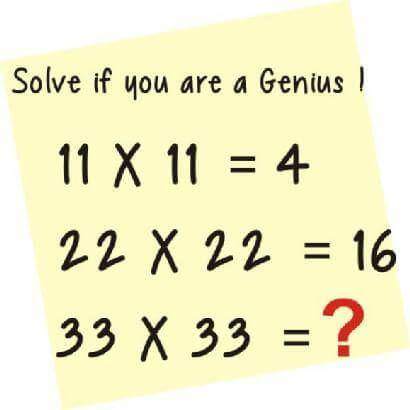Are You a Genius? Solve This

Most people will fail to give the correct answer to this question. If you can solve this, then you are truly a Genius.Related Posts

Corps Member Dies Eight Months After Wedding

36, ama genius
Follow
•      9 months ago
• UGOMEK: 36
9 months ago
• Promise1617: 64 is the answer
9 months ago
• panaye: 36 nd 64
9 months ago
• Kehinde: 1089 is the answer
4 months ago
• olayinkaakindunjoye: 256 is the answer
4 months ago
• pattygal: 36 is the answer

is calculated as 6×6
4 months ago
• udeokwor: the ans is
64 or 256
4 months ago
• Joy: The answer is 18
4 months ago
• sadducees: 1+1=2, 1+1=2, thfor 2x2=4. Now 3+3=6, 3+3=6 so thfor 6×6= ( 36 )
4 months ago
•      4 months ago
• eleazer: 36
4 months ago
•      4 months ago
• Oliver: 36
4 months ago
• lawal ihima musa: 64 is the correct answer
4 months ago
• Magdalene: 64
4 months ago
• Evandro: 36
4 months ago
• Faithwin: 36
4 months ago
• chika: 256
4 months ago
•      4 months ago
• taetifulv: 66 or 36
4 months ago
•      4 months ago
• Nancy: 36
4 months ago
•      4 months ago
• vivian omole: viviant:36
4 months ago
• binahmrabure: 11×11=4 {1+1=2; 1+1=2; 2×2=4}
22×22=6 {2+2=4;2+2=4;4×4=16}
33×33=? {3+3=6;3+3=6;6×6=36}
Ans: 36
1 month ago
1 month ago
• Emmanuel: 36
1 month ago
•      1 month ago
• Samatuto: The correct answer of 33*33 is 36 because 3+3=6 therefore 6*6=36
1 month ago
• Emmanuel: 33
1 month ago
•      1 month ago
• Super babe: It is 36 because... 33*33 equal 1089.. now 1089/30 will give 36. This is likewise to the other ones being solved.
1 month ago
•      1 month ago
• Evelyn: 3+3=6 ,3+3=6
Thus,6×6=36
1 month ago
• Shofunwa: The answer is 36
1 month ago
36
Follow
11*11=1*[1{+}1]=1+1=2
2+1*[1{+}1]=
2+1+1=4
22*22=2*[2{+}2]=4+4=8 =8+2[2{+}2]
=8+8=16
33*33=3*[3{+}3]=9 +9= 18. 18+3*[3{+}3]= 18+9+9=18+18=36
Follow
18
Follow
22*22=484 4+8+4=16
33*33=1089 1+0+8+9=18

dats tge trick guys9 months ago
• esthilo: 256 is the answer
9 months ago
• Isaac: 36
9 months ago
18
Follow
Funny how different methods can give the same answer. Mine is

1^1+1^1+1^1+1^1=4

2^2+2^2+2^2+2^2=16

3^3+3^3+3^3+3^3=36

Lol...
Follow
36
Follow
the ans is 64
Follow
256
4 x 4 = 16
then 16 x 16 = 256
quantitative reasoning
Follow
• Mikejet: The answer is 36
9 months ago
4 months ago
if 11×11 which is (1+1)×(1+1)=4
and 22×22 which is (2+2)×+(2+2)=16
then, 33×33 which is (3+3)×(3+3)= 9×9
=81
therefore, 33×33=81
Follow
• hardeysholar: 11 * 11 = (1+1) * (1+1) which is equal to 2 * 2 = 4 ...same goes for 22 * 22, which is equal to 16 ....same goes for 33 * 33 = (3+3) * (3+3) and its equal to 6 * 6 = 36
4 months ago
Follow
81
Follow
• Movic: 36 sorry
9 months ago
11×11=4
Solution:
1×1+1×1+1×1+1×1=1+1+1+1=4
(2)22×22=16
Solution:
2×2+2×2+2×2+2×2=4+4+4+4=16
(3) 33×33=?
Solution:
3×3+3×3+3×3+3×3=9+9+9+9=36.
I'm a genious too
Follow
No ypu can't dear,to study biology,you need chemistry,biology,maths,english,and agricultural science and any other subject
Follow
the ans is 36 dont av tym to explain
Follow

Quick Questions

• 4 Answers   ·   5 hours ago
• 2 Answers   ·   4 hours ago
• 3 Answers   ·   4 hours ago

Post Comment

Please don't post or ask to join a "Group" or "Whatsapp Group" as a comment. It will be deleted. To join or start a group, please click here

if 11×11 which is (1+1)×(1+1)=4
and 22×22 which is (2+2)×+(2+2)=16
then, 33×33 which is (3+3)×(3+3)= 9×9
=81
therefore, 33×33=81
ABDULJEREMIAH

4 Members
• 22nd
Jun 2019